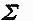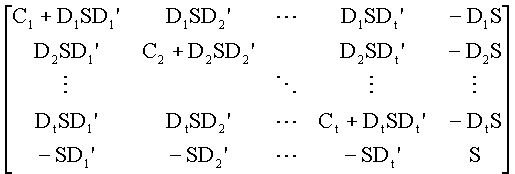The FKF formulas

The FKF formulas for computing state parameters repeatedly (t = 1, 2, 3,... ) are obtained by an augmentation from the Helmert-Wolf formulas as follows:

st = (X'tXt)-1X't(Yt - Gtct)   ... i.e. Weighted Averages (WA) of calibrated data
ct = (G'iRiGi)-1G'iRi Yi   ..... Moving Averages (MA) of computed residuals
where
st = computed state parameters at time t
Yt = vector of normalized observational data, augmented by state parameter estimates or forecasts
Xt = augmented Jacobian matrix for the state parameters
Gt = augmented Jacobian matrix for the calibration or other common state parameters (e.g. Common Factors)
ct = computed calibration and other common state parameters (e.g. scores of the Common Factors)= summation where the index i runs over several time blocks of data
Ri = I-Xi(X'iXi)-1X'i = residual operator generating an "innovation sequence" for time i.

Error estimates of the state vectors s1, s2,…, st, for example, and of the calibration vector ct are obtained by computing the large covariance matrix:where, for i = 1, 2,…, t:
Ci = (X'iXi)-1
Di = (X'iXi)-1X'iGi
S = (G'iRiGi)-1
and, in particular,

Cov(st) = Ct + DtSDt'
Cov(ct) = S.

This extension of the Helmert-Wolf formulas to Fast K- Filtering (FKF) was first reported in Lange, Antti A. (1989): "An Algorithmic Approach for Improving and Controlling the Quality of Upper-Air Data." WMO Instruments and Observing Methods Report, No. 35, World Meteorological Organization, Geneva, Switzerland, 1989, see page 5; and,
thereafter published both in Lange, Antti A. (1990a): "Real-time Optimum Calibration of large sensor systems by K- Filtering." IEEE PLANS '90 - Position Location and Navigation Symposium Record, March 20-23, 1990, pp. 146-149; and,
in Lange, Antti A. (1990b): "Apparatus and method for calibrating a sensor system." International Application Published under the Patent Co-operation Treaty (PCT), World Intellectual Property Organization, International Bureau, WO 90/13794, PCT/FI90/00122, 15 November 1990. See also Lange (1993 and 1997).

The formula for computing the large covariance matrix above was first published in Lange (1982) “Multipath propagation of VLF Omega signals”, IEEE PLANS '82 - Position Location and Navigation Symposium Record, December 1982, see pages 302-309 (308). The recursive FKF solution requires these error covariances in real-time for weighting different data correctly in various mobile (or kinematic) positioning, navigation and process control applications, see Statistical Calibration of Observing Systems by Lange (1999) or more recent discussions in Lange (2000 or 2001).

The FKF method makes it possible to exploit Rao's MINQUE (Minimun Norm Quadratic Unbiased Estimation) theory for the most reliable operational estimation of accuracies of the state and calibration parameters by using smallest low-powered processors. A derivation of these FKF formulas including the large covariance matrix above is given in blackboard snapshots 1, 2 and 3.

Over Internet Customers Served

Sor Etude No8
Sequence © Pierre R. Schwob - by permission. Original from the Classical MIDI Archives
Back to FKF.net
* Last revised January 19, 2002.